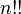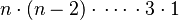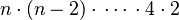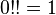# Double factorial facts for kids

Kids Encyclopedia Facts

Double factorial is a method of calculating how many times a number is repeated in a geometric equation. This way, we can calculate the number of times a product is used in its life-time.

The double factorial of n is written as$n!!$. When n is a positive odd integer,$n!!$ is defined as$n \cdot (n-2) \cdot\, \cdots \, \cdot 3 \cdot 1$. When n is an positive even integer,$n!!$ is defined as$n \cdot (n-2) \cdot\, \cdots \, \cdot 4 \cdot 2$. By definition,$0!! = 1$.

## Related pagesDouble factorial Facts for Kids. Kiddle Encyclopedia.